# Basic maths-calculus-Q7

+1 vote

The point on the curve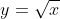which is closest to the point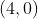is

(A)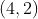(B)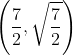(C)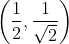(D)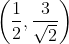asked May 19
reshown May 24

Distance of (4,0) from any point (x,y) is

From the above options, only A,B,C points are the part of curve , now find distance from (4,0) to these options A, B, C . hence correct answer is B.answered May 27 by (112,390 points)
can you please tell formal solution apart from substitution and elimination method
wait I will give you solution. The line from the point(4,0) to the given curve must be a normal to the curve as perpendicular line have the shortest length. So we will use the same concept here. (m1m2 = -1)
+1 vote

This might help with the solutionanswered May 28 by (7,650 points)